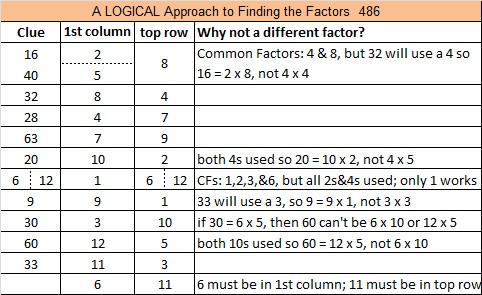# Finding √486 and Level 6

Contents

### Today’s Puzzle:Print the puzzles or type the solution on this excel file: 12 Factors 2015-05-04

Here is a logical order to use the clues to find the solution to the puzzle:### How to Simplify the Square Root of 486:

To find √486, I like to first see if 486 can be evenly divided by square numbers 4 or 9 because about 82% of numbers that can have their square roots reduced are divisible by 4 and/or by 9. It is also very easy to tell if a number can be evenly divided by 4 or by 9.

86 is not divisible by 4, so 486 is not divisible by 4.

However, 4 + 8 + 6 = 18, a multiple of 9 so 486 is divisible by 9, and now we know for sure that its square root can be simplified. 486 ÷ 9 = 54, another multiple of 9 so we get this two-layer cake when we divide by 9 twice.Now take the square root of everything on the outside of the cake, and we get √486 = (√9)(√9)(√6) = 9√6.

### Factors of 486:

• 486 is a composite number.
• Prime factorization: 486 = 2 x 3 x 3 x 3 x 3 x 3, which can be written 486 = 2 x 3⁵.
• The exponents in the prime factorization are 1 and 5. Adding one to each and multiplying we get (1 + 1)(5 + 1) = 2 x 6 = 12. Therefore 486 has exactly 12 factors.
• Factors of 486: 1, 2, 3, 6, 9, 18, 27, 54, 81, 162, 243, 486
• Factor pairs: 486 = 1 x 486, 2 x 243, 3 x 162, 6 x 81, 9 x 54, or 18 x 27
• Taking the factor pair with the largest square number factor, we get √486 = (√81)(√6) = 9√6 ≈ 22.04540### Sum-Difference Puzzles:

6 has two factor pairs. One of those pairs adds up to 5, and the other one subtracts to 5. Put the factors in the appropriate boxes in the first puzzle.

486 has eight factor pairs. One of the factor pairs adds up to 45, and a different one subtracts to 45. If you can identify those factor pairs, then you can solve the second puzzle!The second puzzle is really just the first puzzle in disguise. Why would I say that?

## 2 thoughts on “Finding √486 and Level 6”

1.Paula Beardell Krieg

82% of numbers that can have their square roots reduced are divisible by 4 and/or by 9? Now that’s a fact one doesn’t come by every day! That rather boggles my mind.

•ivasallay

Hardly anyone is aware of this fact, too. Those who know it can have an advantage over those who don’t anytime a square root needs to be reduced.

This site uses Akismet to reduce spam. Learn how your comment data is processed.